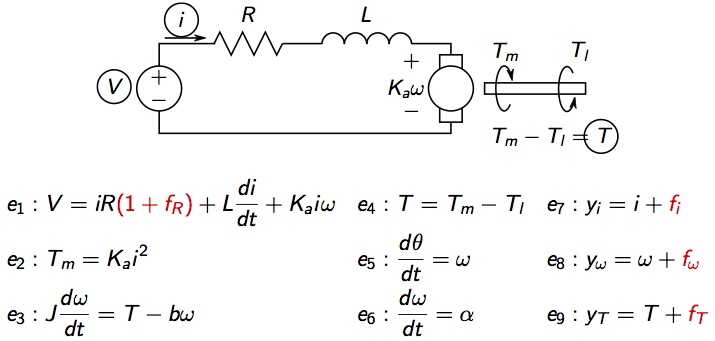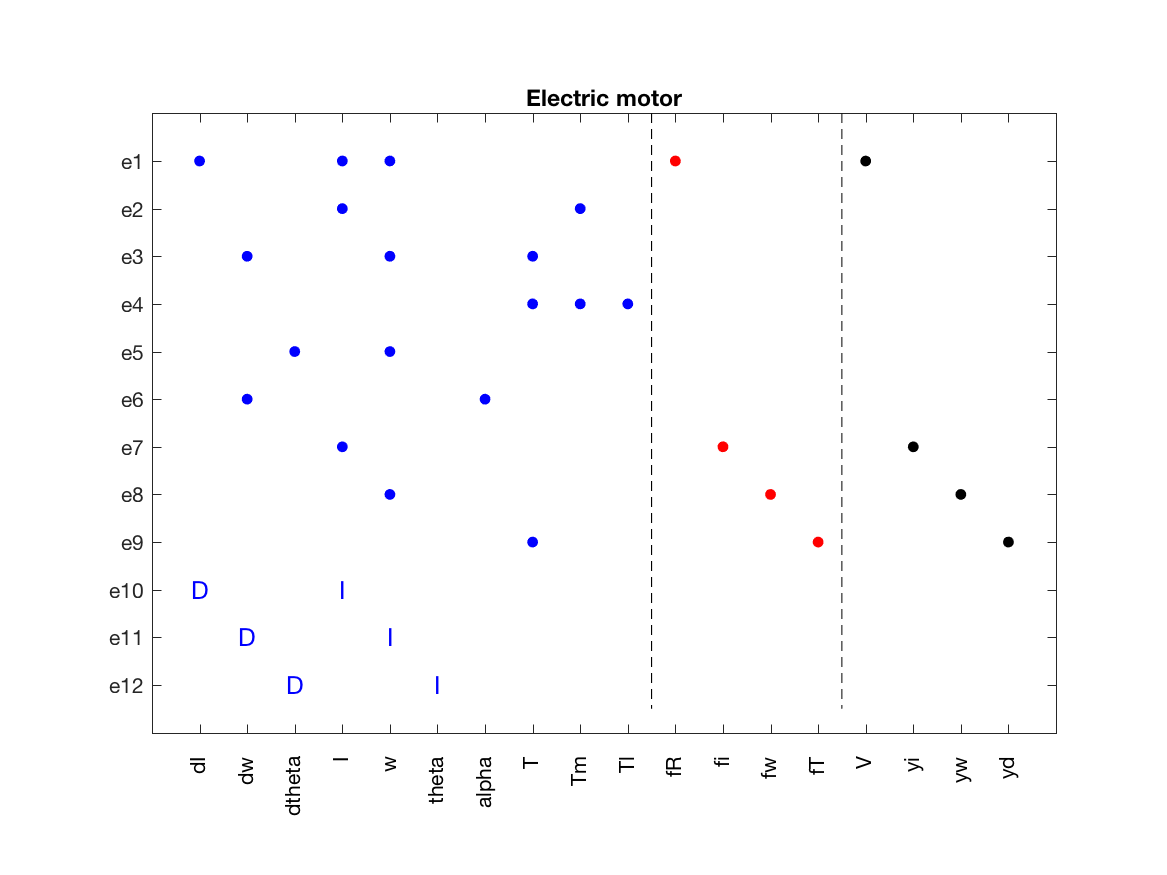# Defining a symbolic dynamic model

04 Mar 2017

This tutorial describes how to define a symbolic model with dynamic constraints.

Again, as in a previous tutorial an idealized model of an electric motor will be usedwhere V is voltage, i current, Tm motor torque, Tl load torque, omega rotational speed, theta motor angle. There are four considered faults, three sensor faults and a change in the internal resistance.

Here the full symbolic model, including dynamic constraints, will be defined. For this, create a model definition with the type

After that, partition the set of variables in three categories; unknown (x), known (z), and faults (f), and also determine the set of parameters in the model. Since we are considering dynamics, introduce variables also for the derivatives. Then,

The next step is to define symbolic expressions for all model variables so that the symbolic toolbox can manage them.

Now, we can state the model equations directly as

Note especially how the differential constraints e10, e11, and e12 are defined. Also, you can clean up the workspace to keep it from cluttering by

Now, the model definition is complete and we can create the model object and give it a name as before

With the model object defined, print some basic model information using the class method `Lint`

The model structure is automatically inferred from the analytical expressions. And to graphically plot the model structure, use the `PlotModel` class method

which produces the figureHere, the dynamic constraints can be seen as I and D edges in the incidence matrix.

Categories:

Updated: